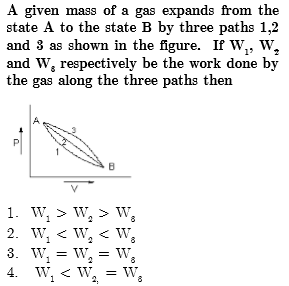Concept Videos :-

#19 | Work (W) done by the Gas: 1
#20 | Solved Example: 7
#21 | Work (W) done by the Gas: 2
#22 | Solved Example: 8
#23 | Solved Example: 9
#24 | Work (W) done by the Gas: 3
#25 | Graphical Intrepretation of Work done
#26 | Solved Example: 10
#27 | Solved Example: 11
#28 | Solved Example: 12

Concept Questions :-

Work done by gas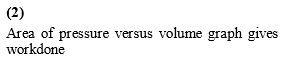Difficulty Level:

• 15%
• 54%
• 28%
• 5%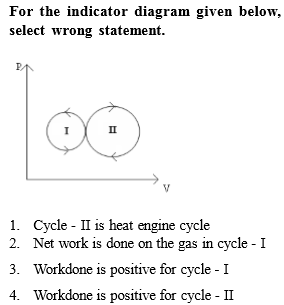Concept Videos :-

#19 | Work (W) done by the Gas: 1
#20 | Solved Example: 7
#21 | Work (W) done by the Gas: 2
#22 | Solved Example: 8
#23 | Solved Example: 9
#24 | Work (W) done by the Gas: 3
#25 | Graphical Intrepretation of Work done
#26 | Solved Example: 10
#27 | Solved Example: 11
#28 | Solved Example: 12

Concept Questions :-

Work done by gas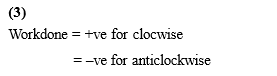Difficulty Level:

• 7%
• 16%
• 49%
• 30%

The P-V diagram for a thermodynamic system is shown in the figure. The work done by the system during the cyclic process ABCA is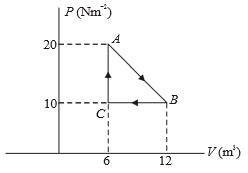1.  Zero

2.  90 J

3.  30 J

4.  60 J

Concept Videos :-

#19 | Work (W) done by the Gas: 1
#20 | Solved Example: 7
#21 | Work (W) done by the Gas: 2
#22 | Solved Example: 8
#23 | Solved Example: 9
#24 | Work (W) done by the Gas: 3
#25 | Graphical Intrepretation of Work done
#26 | Solved Example: 10
#27 | Solved Example: 11
#28 | Solved Example: 12

Concept Questions :-

Work done by gas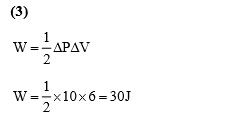Difficulty Level:

• 16%
• 13%
• 62%
• 11%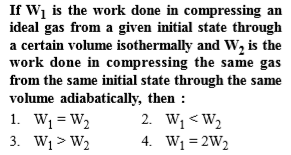Concept Videos :-

#19 | Work (W) done by the Gas: 1
#20 | Solved Example: 7
#21 | Work (W) done by the Gas: 2
#22 | Solved Example: 8
#23 | Solved Example: 9
#24 | Work (W) done by the Gas: 3
#25 | Graphical Intrepretation of Work done
#26 | Solved Example: 10
#27 | Solved Example: 11
#28 | Solved Example: 12

Concept Questions :-

Work done by gas

For compression:-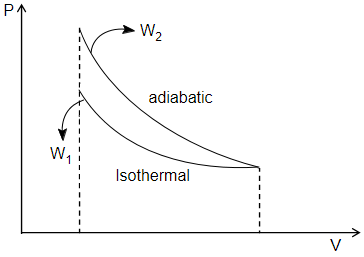Work done = area under P - V curve.

Work done is more for adiabatic compression.

Difficulty Level:

• 13%
• 54%
• 32%
• 3%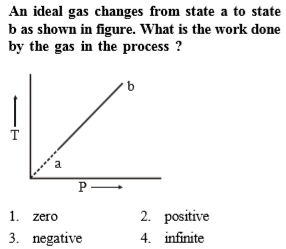Concept Videos :-

#19 | Work (W) done by the Gas: 1
#20 | Solved Example: 7
#21 | Work (W) done by the Gas: 2
#22 | Solved Example: 8
#23 | Solved Example: 9
#24 | Work (W) done by the Gas: 3
#25 | Graphical Intrepretation of Work done
#26 | Solved Example: 10
#27 | Solved Example: 11
#28 | Solved Example: 12

Concept Questions :-

Work done by gas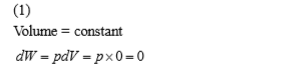Difficulty Level:

• 44%
• 38%
• 13%
• 7%

A gas is compressed isothermally to half its initial volume. The same gas is compressed separately through an adiabatic process until its volume is again reduced to half .Then -

(a) compressing the gas through adiabatic process will require more work to be done
(b) compressing the gas isothermally or adiabatically will require the  same amount work
(c) which of the case (whether compression through isothermal or through adiabatic process) requires more work will depend upon the atomicity of the gas
(d) compressing the gas isothermally will require more work to be done

Concept Videos :-

#19 | Work (W) done by the Gas: 1
#20 | Solved Example: 7
#21 | Work (W) done by the Gas: 2
#22 | Solved Example: 8
#23 | Solved Example: 9
#24 | Work (W) done by the Gas: 3
#25 | Graphical Intrepretation of Work done
#26 | Solved Example: 10
#27 | Solved Example: 11
#28 | Solved Example: 12

Concept Questions :-

Work done by gas

(a) The solution of this question can be understood by plotting a p-V graph for the compression of a gas isothermally and adiabatically simultaneously to half of its initial volume, ie.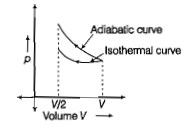Since, the isothermal curve is less steeper than the adiabatic curve. So. area under the p-V curve for adiabatic process has more magnitude than isothermal curve. Hence, work done in adiabatic process will be more than in isothermal process

Difficulty Level:

• 52%
• 18%
• 16%
• 17%

An ideal gas is compressed to half its initial volume by means of several process. Which of the process results in the maximum work done on the gas?

(b) Isobaric

(c) Isochoric

(d) Isothermal

Concept Videos :-

#19 | Work (W) done by the Gas: 1
#20 | Solved Example: 7
#21 | Work (W) done by the Gas: 2
#22 | Solved Example: 8
#23 | Solved Example: 9
#24 | Work (W) done by the Gas: 3
#25 | Graphical Intrepretation of Work done
#26 | Solved Example: 10
#27 | Solved Example: 11
#28 | Solved Example: 12

Concept Questions :-

Work done by gas

Given, ideal gas is compressed to half its inital volume i.e
Vo=V/2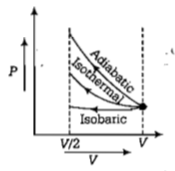The isochoric process is one in which volume is kept constant, meaning that work done by the system will be zero. i.e. Wisochoric=0

As we know, work done on the gas=Area under the curve i.e.

Difficulty Level:

• 64%
• 19%
• 9%
• 10%

A gas is taken through the cycle A→B→C→A, as shown. What is the net work done by the gas?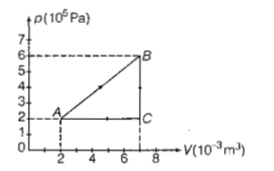(a)2000J

(b)1000J

(c)Zero

(d)-2000J

Concept Videos :-

#19 | Work (W) done by the Gas: 1
#20 | Solved Example: 7
#21 | Work (W) done by the Gas: 2
#22 | Solved Example: 8
#23 | Solved Example: 9
#24 | Work (W) done by the Gas: 3
#25 | Graphical Intrepretation of Work done
#26 | Solved Example: 10
#27 | Solved Example: 11
#28 | Solved Example: 12

Concept Questions :-

Work done by gas

(b) Net work done=Area enclosed in pV curve,i.e., ΔABC
=1/2 x 5 x 10-3 x 4 x 105 J

=103 J

=1000

Difficulty Level:

• 14%
• 56%
• 26%
• 6%

A cylinder fitted with a piston contains 0.2 moles of air at temperature 27°C. The piston is pushed so slowly that the air within the cylinder remains in thermal equilibrium with the surroundings. Find the approximate work done by the system if the final volume is twice the initial volume

(1) 543 J

(2) 345 J

(3) 453 J

(4) 600 J

Concept Videos :-

#19 | Work (W) done by the Gas: 1
#20 | Solved Example: 7
#21 | Work (W) done by the Gas: 2
#22 | Solved Example: 8
#23 | Solved Example: 9
#24 | Work (W) done by the Gas: 3
#25 | Graphical Intrepretation of Work done
#26 | Solved Example: 10
#27 | Solved Example: 11
#28 | Solved Example: 12

Concept Questions :-

Work done by gas

(2) $W=\mu RT{\mathrm{log}}_{e}\left(\frac{{V}_{2}}{{V}_{1}}\right)$

$=0.2×8.3×{\mathrm{log}}_{e}2×\left(27+273\right)$

$=0.2×8.3×300×0.693=345J$

Difficulty Level:

• 12%
• 59%
• 20%
• 10%

Two identical samples of a gas are allowed to expand (i) isothermally (ii) adiabatically. Work done is

(1) More in the isothermal process

(2) More in the adiabatic process

(3) Neither of them

(4) Equal in both processes

Concept Videos :-

#19 | Work (W) done by the Gas: 1
#20 | Solved Example: 7
#21 | Work (W) done by the Gas: 2
#22 | Solved Example: 8
#23 | Solved Example: 9
#24 | Work (W) done by the Gas: 3
#25 | Graphical Intrepretation of Work done
#26 | Solved Example: 10
#27 | Solved Example: 11
#28 | Solved Example: 12

Concept Questions :-

Work done by gas

(1) In thermodynamic processes.

Work done = Area covered by PV diagram with V-axis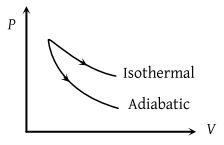From graph it is clear that ${\text{(Area)}}_{\mathrm{iso}}>{\left(\mathrm{Area}\right)}_{\mathrm{adi}}$

${W}_{iso}>{W}_{adi}$

Difficulty Level:

• 50%
• 43%
• 5%
• 4%